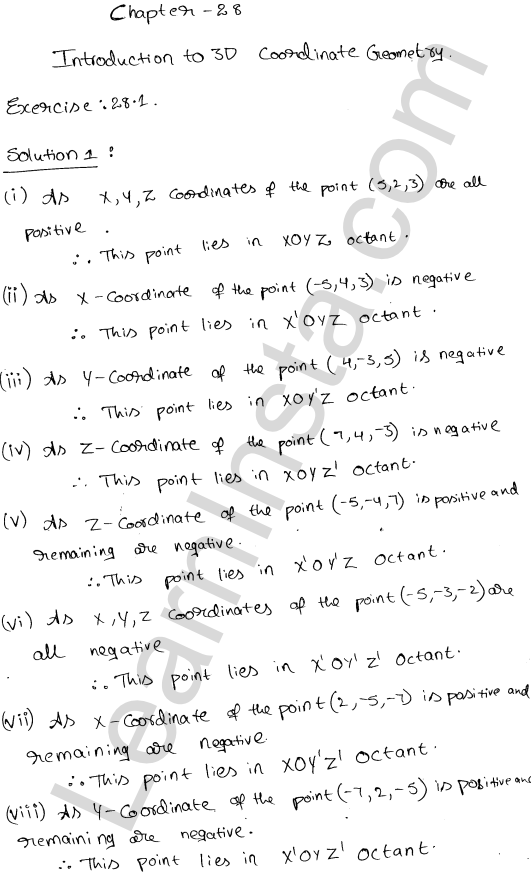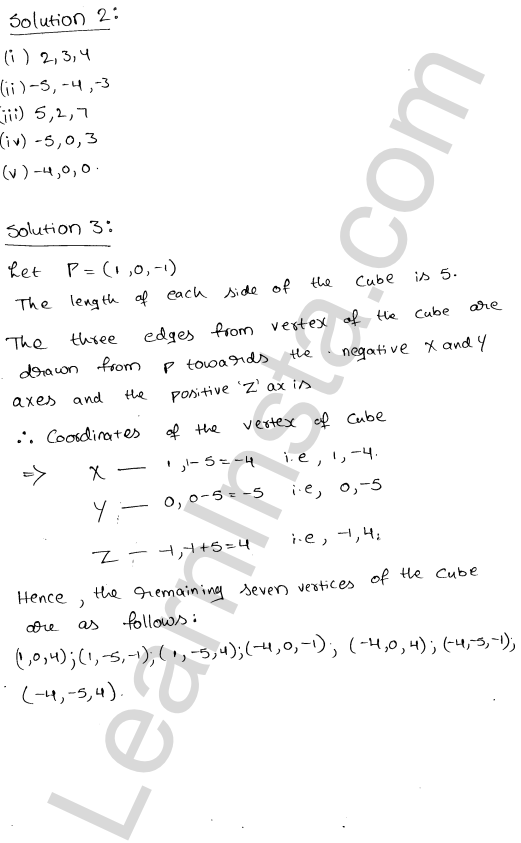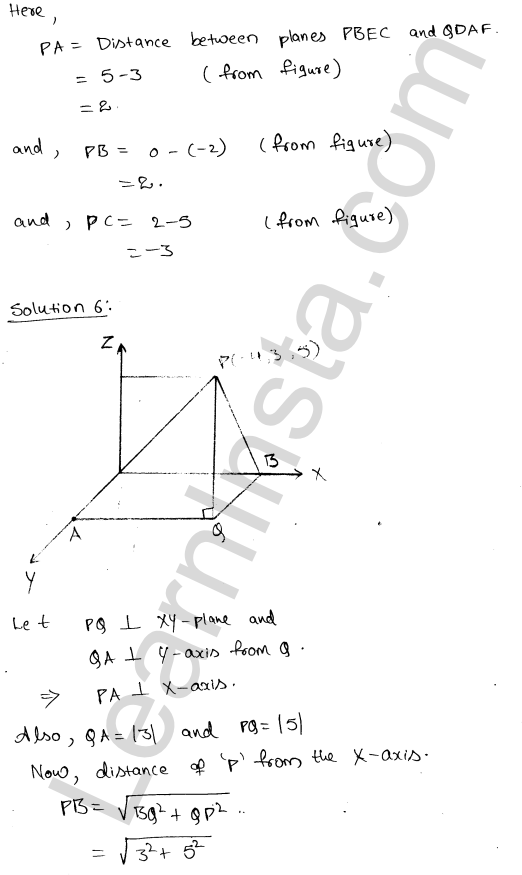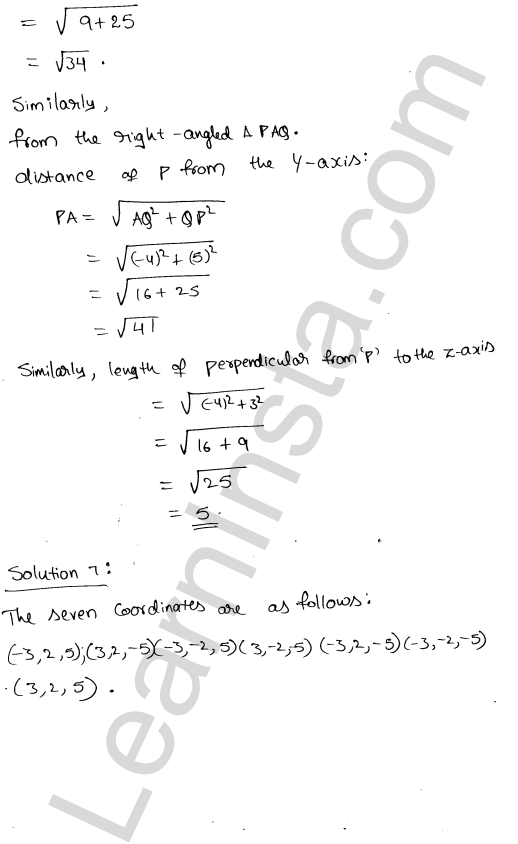# RD Sharma Class 11 Solutions Chapter 28 Introduction to 3D coordinate geometry Ex 28.1

## RD Sharma Class 11 Solutions Chapter 28 Introduction to 3D coordinate geometry Ex 28.1

These Solutions are part of RD Sharma Class 11 Solutions. Here we have given RD Sharma Class 11 Solutions 28 Introduction to 3D coordinate geometry Ex 28.1.We hope the RD Sharma Class 11 Solutions Chapter 28 Introduction to 3D coordinate geometry Ex 28.1, help you. If you have any query regarding RD Sharma Class 11 Solutions Chapter 28 Introduction to 3D coordinate geometry Ex 28.1, drop a comment below and we will get back to you at the earliest.